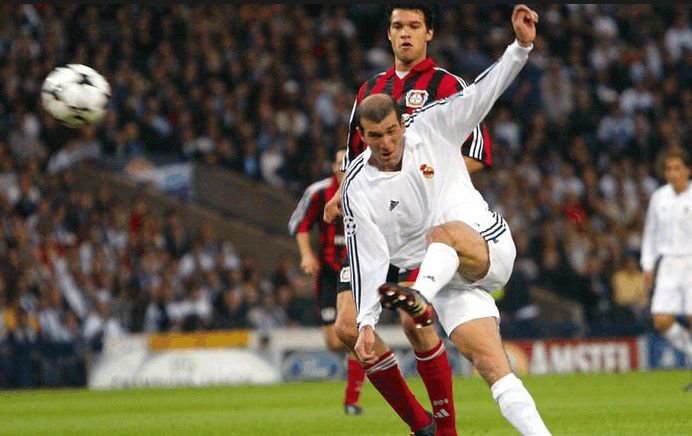• [ 宝应县 ]
• [ 高邮市 ]
• [ 仪征市 ]
• [ 江都区 ]
• [ 邗江区 ]
• [ 广陵区 ]
• [ 开发区 ]
• [ 化工园区 ]
• [生态科技新城]
• [ 蜀冈瘦西湖景区 ]
• [ 发改委 ]
• [ 教育局 ]
• [ 科技局 ]
• [ 工信局 ]
• [民宗局]
• [ 公安局 ]
• [ 民政局 ]
• [ 司法局 ]
• [ 财政局 ]
• [ 人社局 ]
• [ 自然资源局 ]
• [ 生态环境局 ]
• [ 住建局 ]
• [ 城管局 ]
• [ 交通局 ]
• [ 水利局 ]
• [ 农业农村局 ]
• [ 商务局 ]
• [文化广电和旅游局]
• [ 卫健委 ]
• [ 退役军人事务局 ]
• [ 应急管理局 ]
• [ 国资委 ]
• [ 政务办 ]
• [ 市场监管局 ]
• [ 体育局 ]
• [ 统计局 ]
• [ 医保局 ]
• [ 金融监管局 ]
• [ 机关事务局 ]
• [ 税务局 ]
• [ 残联 ]
• [ 邮政管理局 ]
• [ 公积金中心 ]
• [ 银保监分局 ]
• [城建控股]
• [交通产业集团]
• [有线电视公司]
• [供电公司]
• [广电总台]
• [  其他  ]
 网友: 城市观察员 时间: 2020-09-21 部门: 高邮市 类别: 建议 尊敬的领导好！高邮正在创文明城市，(欧洲城一期)小区门口整治还看的过去(表面文章？)，小区里面及楼道还是跟往常一样(垃圾桶东倒西歪、垃圾、旧杂物到处可见)；小区能整治好也是创建文明城市的一部分，我们非常支持和协助，我们也看过很多小区(欧洲城一期小区服务与管理大概是全市最差的)，希望领导亲临关注、关注，谢谢领导！